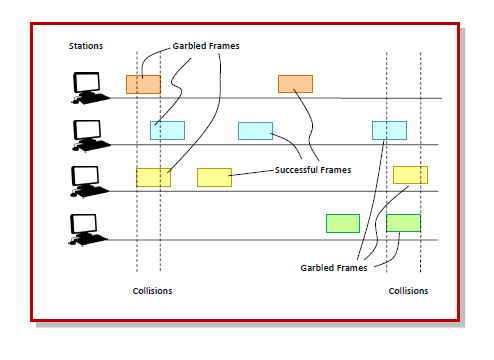# Pure ALOHA

ALOHA is a medium access control (MAC) protocol for transmission of data via a shared network channel. Using this protocol, several data streams originating from multiple nodes are transferred through a multi-point transmission channel. There are two types of ALOHA protocols – Pure ALOHA and Slotted ALOHA.

In pure ALOHA, the time of transmission is continuous. Whenever a station has an available frame, it sends the frame. If there is collision and the frame is destroyed, the sender waits for a random amount of time before retransmitting it.

## Working Principle

After transmitting a frame, a station waits for a finite period of time to receive an acknowledgement. If the acknowledgement is not received within this time,the station assumes that the frame has been destroyed due to collision and resends the frame.

A collision occurs if more than one frame tries to occupy the channel at the same time. The situation is depicted in the following diagram−## Throughput of Pure ALOHA

Let 𝑇 be the frame time, i.e. the time required for 1 frame to be transmitted.

Let G be the number of transmission attempts per frame time.

The probability that 𝑘 frames are generated during the frame time is given by the Poisson distribution−

$$P(k)=\frac{G^k e^{-G}}{k!}$$

From this we can say that the probability that 0 frames are generated ( 𝑘k = 0 ) during the frame time is $e^{-G}$.

In case of pure ALOHA, the vulnerable time period so that collision does not occur between two frames is equal to two frame times, i.e. 2T𝑇. In 2T time,average number of transmission attempts is 2G.

The probability that 0 frames are initiated in the vulnerable time period will be −

$$P(0) = e^{-2G}$$.

The throughput, 𝑆, is calculated as the number of transmission attempts per frame time, 𝐺, multiplied by the probability of success, 𝑃(0).

$$S = G.P(0)$$

$$S = G.e^{-2G}$$

## Maximum Throughput of Pure ALOHA

The maximum throughput occurs when G𝐺=0.5.

The maximum throughput is thus−

$S_{max}$𝑎𝑥 = $0.5𝑒e^{-2*0.5}$ = $\frac{1}{2e}= 0.184$

Thus, it can be seen that the maximum throughput is only 18.4% in pure ALOHA.